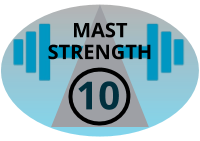## Strength Rating System for Telescopic Fibreglass Masts

Posted by Richard Newstead on 3rd Oct 2018People often ask me how mast X compares with mast Y in terms of strength and flexibility. It's hard to be objective but in an attempt to do so, I have developed a simple rating system.

The SOTABEAMS Mast Rating System (SBMRS) uses the length of the mast (in metres) and its weight in kilograms. It is only applicable to telescopic masts made of fibreglass.

The SBMRS is calculated as follows:

10+(10.Log((m/L)/0.2357))

Where L is the extended mast length in metres, m is the mass in kg.

Log is Log base 10.

Some examples:

SOTABEAMS Tactical 7000hds L=7 m=1.65

SBMRS = 10

SOTABEAMS SOTAPOLE 7m L=7 m=0.65

SBMRS = 6

SOTABEAMS SOTAPOLE 10m L=10 m=1.4

SBMRS = 7.7

SOTABEAMS Travel Mast L=10 m=1.3

SBMRS = 7.4

SOTABEAMS Mini Pole L=4.1 m=0.3

SBMRS = 4.9

Spiderbeam 18m L=18 m=6.8

SBMRS = 12

Spiderbeam 26m L=26 m=16

SBMRS = 14.7

The higher the SBMRS the stronger/stiffer the mast but the less suitable it will be for lightweight portable use.